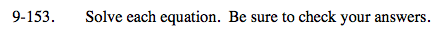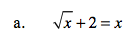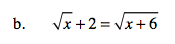### Home > A2C > Chapter Ch9 > Lesson 9.3.2 > Problem9-153

9-153.Square both sides.

Set the equation equal to 0.

Factor the equation.

$\sqrt{\textit{x}}=\textit{x}-2$

x = x2 − 4x + 4

x2 − 5x + 4 = 0

(x − 4)(x − 1)

x = 4You will need to square twice.